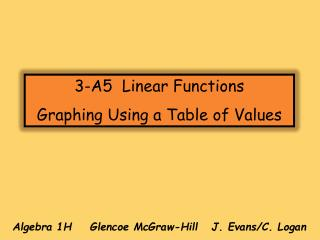# Algebra 1H Glencoe McGraw-Hill J. Evans/C. Logan - PowerPoint PPT PresentationDownload PresentationAlgebra 1H Glencoe McGraw-Hill J. Evans/C. Logan

Algebra 1H Glencoe McGraw-Hill J. Evans/C. LoganDownload Presentation## Algebra 1H Glencoe McGraw-Hill J. Evans/C. Logan

- - - - - - - - - - - - - - - - - - - - - - - - - - - E N D - - - - - - - - - - - - - - - - - - - - - - - - - - -
##### Presentation Transcript

1. 3-A5 Linear Functions Graphing Using a Table of Values Algebra 1H Glencoe McGraw-Hill J. Evans/C. Logan

2. In Chapter 2 you solved linear equations. In a linear equation the exponent of the variable is one. 1 In this lesson you will graph linear equations in two variables. In a linear equation with two variables the exponent of the variables is one (or zero). 1 1

3. In this lesson the equations will each have TWO VARIABLES, x and y The graph of a linear equation is the collection of all points (x, y) that are SOLUTIONS of the equation. How many points will the graph of a line contain? Way too many points to list.

4. Make a table of values (using advantageous x-values). • Graph enough points from the table to recognize a pattern. 3. Connect the points to form a line.

5. Ex. 1: Graph y = 2x + 3 by constructing a table of values and graphing the solutions. Describe the pattern you notice. y x y = 2(-3) + 3 = -3 x y -3 -2 -1 0 1 ( ) -3 ( ) -1 ( ) 1 The pattern? The points all lie on a line. The ENTIRE line, even the parts not shown, is the graph of y = 2x + 3. Every point on the line is a solution to the equation y = 2x + 3. ( ) 3 ( ) 5

6. Before sketching a graph, make sure your equation is in “function form”. In function form, the y is isolated, making it much easier to construct a table of values.

7. Think of an equation in function form as a type of machine……a function machine. The function machine changes numbers. The input(the x value) enters the function machine and the function produces an output (the y value). Input thex y is the output

8. xy -3 -2 -1 0 1 2 Substitute the x values to find the corresponding values for y.

9. y xy -3 -2 -1 x 0 1 2

10. xy -4 -2 0 2 4 What xvalues should you choose?

11. y xy -4 -2 0 x 2 4

12. xy -2 -1 0 1 2 What do you need to do first?

13. x y y -2 -1 0 1 x 2 (2, 13) will be off the graph. Four points should be sufficient.

14. Important!! When you plot the points on the graph they should lie in a straight line. These are linear equations. If the points you plot don’t lie in a straight line you have either made an arithmetic mistake when you substituted in the x values -or- you have plotted the points incorrectly! Check your work to find the mistake—don’t draw a crooked line!

15. No graphs will be accepted if they have not been neatly and carefully drawn on graph paper with a straight edge. This is non-negotiable!